## R-USERS-L@LISTS.UFL.EDU

#### View:

 Message:[First|Previous|Next|Last] By Topic:[First|Previous|Next|Last] By Author:[First|Previous|Next|Last] Font: Proportional Font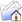LISTSERV Archives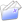R-USERS-L HomeR-USERS-L 2018

Subject:Re: Help with code: lm in a loop

From:Date:Wed, 16 May 2018 19:07:37 +0000

Content-Type:text/plain

Parts/Attachments: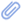text/plain (108 lines)
 ```You need to create an empty "test" vector beforehand, and in each loop you need to append the "e" to the "test" variable for it to work. The code might look something like this: test <- c() for (s in sub) {   ...   test <- rbind(test, e) } This way, "test" will be a matrix of 48 rows and n columns by the end of the loop. The major caveat of using this method is, in each loop (i.e. each regression fit) you must have exactly the same number of data point (n). If say loop 1 has 20 points but loop 2 has 25 points, this method will not work. Also if the name of the fitted value is important, this will not work too. If you want your output to be rich, and at the same time avoiding the caveat, you'll need to append a dataframe onto the "test" variable instead of a vector, for example: test <- data.frame() for (s in sub) {   ...   df <- data.frame(loop = s, id = name(e), value = as.vector(e))   test <- rbind(test, df) } Let us know if this helps! Cheers, Ben -----Original Message----- From: UF R Users List <[log in to unmask]> On Behalf Of Haro Carrion, Xavier Sent: Wednesday, May 16, 2018 2:39 PM To: [log in to unmask] Subject: Help with code: lm in a loop Hello all, I usually attend the Wednesdays meetings of this group, so in its absence, I will try this listserv. Sorry to populate your emails. I have the following code that runs, in a for loop, a series of linear regressions of NDVI by time (29 years). I run a total of 48 regressions (each month * 4 locations). Inside the loop, I save some information I need from each regression (i.e. slope, R2, p-value). Then I save that information in a data frame outside the loop. So far, so good. This works quite well. I am trying now to get the fitted values of each point, that is 1392 values (48 regressions, each based on 29 years of data). Here is the code: NOTE: sub is a list of size 48, Reg_summaries a dataframe defined outside the loop for(s in sub){   # Print which row is being calculated   print(s)   # Subset using indexing vector   tmp <- tmp_dt %>%     filter(Triad.Regions == s)   # Run linear model on tmp (this exists only inside the loop)   m <- lm(meanNDVI ~ Year, data = tmp)   # Create dataframe for single loop iteration and saves in the dataframe the data   d <- data.frame(Triad.Regions = s,                   Intercept = as.numeric(coef(m)),                   Slope = as.numeric(coef(m)),                   R2= as.numeric(summary(m)\$r.squared),                   p= as.numeric(summary(m)\$coefficient))   e <- fitted(m)   # Bind temporal dataframe to stored dataframe outside the loop   Reg_summaries <- rbind(Reg_summaries, d)   # Data frame to store fitted values   test <- rbind(e) } I tried to incorporate inside the loop something similar to what I have to get information from the regressions to retrieve the 1392 fitted values. I created "vector e" inside the loop, and various variations of it, nothing seems to work. I created a dataframe of 1392 values outside the loop and called it inside, got an error complaining about column sizes, created a list inside and outside, nothing. With "e" as it is, I get 29 values that get stored in "test", which makes me thing I am getting the 29 fitted values of the first regression, but I don't know how to retrieve them all. Maybe I have to do a loop inside the already existing loop, no clue. Any help will be appreciated. Xavier Xavier Haro-Carrión Ph.D. student School of Natural Resources and Environment & Department of Geography Land Use & Environmental Change Institute (LUECI) University of Florida Biodiversity Institute (UFBI) University of Florida TUR 3141, Gainesville FL 32611 Email: [log in to unmask]; [log in to unmask] This list strives to be beginner friendly. However, we still ask that you PLEASE do read the posting guide http://www.R-project.org/posting-guide.html and provide commented, minimal, self-contained, reproducible code. This list strives to be beginner friendly. However, we still ask that you PLEASE do read the posting guide http://www.R-project.org/posting-guide.html and provide commented, minimal, self-contained, reproducible code.```

#### Options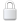Log In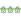Get Password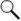Search Archives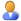Subscribe or Unsubscribe

2020
2019
2018
2017
2016
2015
2014
2013
2012
2011
2010
2009
2008